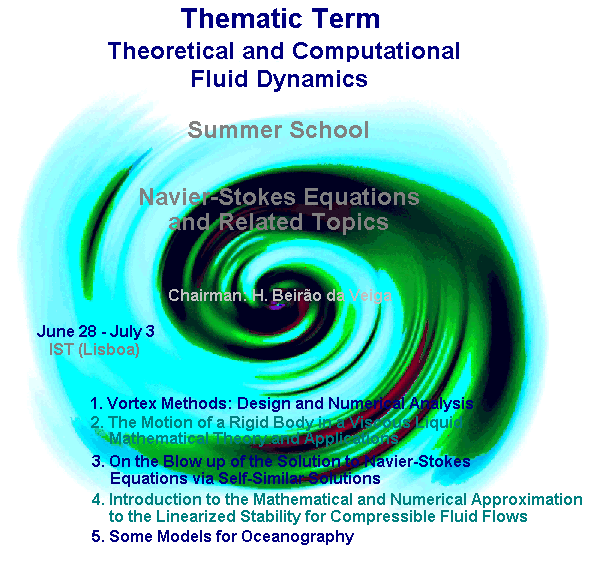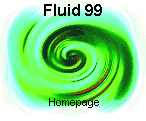Navier-Stokes Equationsand Related Topics

Chairman: H. Beirăo da Veiga
________Centro de Matemática Aplicada
Instituto Superior Técnico
June 28 - July 3, Lisbon (Portugal)

1. On the Blow Up of the Solutions to the Navier-Stokes Equations via Self-Similar Solutions
J. Necas (Charles University, Prague, Czech Republic, and North. Illinois Univ., U.S.A.)

2. The Motion of a Rigid Body in a Viscous Liquid: Mathematical Theory and Applications
G. P. Galdi (Univ. Pittsburgh, U.S.A.)

3. Vortex methods: Design and Numerical Analysis
G. H. Cottet (LMC, Univ. Grenoble I, France)

4. Introduction to the Mathematical and Numerical Approximation to the Linearized Stability for Compressible Fluid Flows
P. A. Raviart (CMAP, Ecole Polytechnique, France)

5. Some Models in Oceanography
L. Tartar (Carnegie Mellon Univ., Pittsburgh, U.S.A.)On the Blow Up of the Solutions to the Navier-Stokes Equations via Self-Similar Solutions

J. Necas
(Charles University, Prague, Czech Republic
and North. Illinois Univ., U. S. A.)

The self-similar solutions, if non-trivial, produce a blow up, as it was proposed by J.Leray in his famous paper in 1934. We shall present several results showing the non-existence of a non-trivial backward self-similar solution for the Cauchy problem. All those results are strongly based on the maximum principle for a special form of the combination of kinetic energy, pressure and momentum of the speed.

Surprisingly, a completely other method, developed for the construction of a non-trivial solution in the half-space will be shown to give the same result.The Motion of a Rigid Body in a Viscous Liquid: Mathematical Theory and Applications

G. P. Galdi
(University of Pittsburgh, U.S.A.)

The problem of the motion of a rigid body through a liquid has attracted the attention of several scientists over a century ago. Actually, the first systematic study was initiated by Kirchhoff and Lord Kelvin in the second half of nineteenth century, in the case when the liquid is frictionless. The main feature of this type of problems relies in the fact that the mechanical system is constituted by the solid and the liquid, so that the motion of the one influences that of the other and vice versa. Typical examples are, for instance, the steady fall of a body in a viscous liquid under the action of gravity, or the selfpropulsion of a micro-organism in an organic suspension. From the mathematical viewpoint, these problems find their natural formulation in the framework of exterior problems. The interesting issue, however, is that here the equations governing the motion of the body are coupled with those of the liquid. This should be contrasted with the "classical" exterior problem, where the motion of the body is prescribed.

The objective of these lectures is to give an introductory course on the above subject, with special emphasis to the case when the liquid is either an incompressible Navier-Stokes fluid or is a second-order approximation of a viscoelastic incompressible fluid. In particular, we shall formulate existence and uniqueness results for initial-boundary as well as boundary-value problems. These results will then be applied to the problem of particle sedimentation and to selfpropulsion of ciliated organisms. We shall also analyze the stability of steady solutions and compare our results with experiments.Vortex Methods: Design and Numerical Analysis.

G.-H. Cottet
(LMC, Univ. Grenoble I, France)

The goal of these lectures is to give an overview of vortex methods with emphasis on some recent developments which make them viable alternative to more comventional methods for Direct Numerical Simulations or Large Eddy Simulations of incompressible flows. The lessons will follow the outline of a book written with P. Koumoutsakos which is coming out in 1999 at Cambridge University Press and will be illustrated with a number of numerical simulations.

Lecture 1:
We present the basic definitions of vortex methods in 2D. We show their conservation properties and illustrate their main features on vortex sheet caluclations.
Lecture 2:
We describe the various 3D algorithms (vortex filament and vortex particle methods), and the available viscous schemes (random walk and deterministic methods).
Lecture 3:
We outline the numerical analysis of vortex methods. The analysis is based on the notion of weak solutions to transport equations and error estimates are derived in distribution spaces.
Lecture 4:
We focus here on boundary conditions. we in particular describe vorticity boundary conditions for viscous flows and their links with vorticity creation algorithms.
Lecture 5:
We present here some developpments of vortex methods towards domain decomposition and hybrid particle-grid techniques. We also show the particular role played by vortex methods in the context of Large Eddy simulations.Introduction to the Mathematical and Numerical Approximation to the Linearized Stability for Compressible Fluid Flows

P. A. Raviart
(Ecole Polytechnique, France)

Stability of flows is a classical problem in Fluid Mechanics. If we neglect dissipative phenomena (viscosity, heat conduction,...), most of fluid flows can be modeled by means of a nonlinear hyperbolic system of conservation laws. We are thus faced to study the stability of the solutions of these nonlinear systems. Given such a solution, called basic solution, the first step consists in studying its linearized stability, i.e., the behavior in time of solutions of the linearized hyperbolic system obtained by linearizing the system of conservation laws at the basic solution. The course is a mathematical introduction to the linearized stability of solutions of nonlinear hyperbolic systems with applications to compressible fluid flows.

The plan of the course is as follows.

1) The problem of linearized stability; the linearized hyperbolic systems;the case of discontinuous basic solutions.
2) Application to the Euler equations of compressible gas dynamics; the Rayleigh-Taylor and Kelvin-Helmholtz instabilities; the Riemann problem and the Richtmyer-Meshkov instability.
3) The case of high frequency perturbations : the W.K.B. method.
4) A general numerical approach for the linearized stability.Some Models in Oceanography

L. Tartar
(Carnegie Mellon Univ., Pittsburgh, U.S.A.)

The lectures will be concerned with various questions which arise when modeling fluid flows in Oceanography: which variants of Navier-Stokes equation should one consider, which boundary conditions should one use, are examples of these questions.